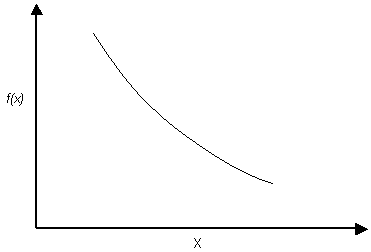Expert Modelling: Hands-on help from Rocscience Engineers. Register Here

# Exponential Distribution

The EXPONENTIAL probability density function is available in Slide:Exponential Probability Density Function

NOTE:

1. The range of values must always be positive for an EXPONENTIAL distribution. It must therefore not be used for random variables which include negative values.
2. The mean of an EXPONENTIAL distribution is equal to its standard deviation. This is a property of the EXPONENTIAL distribution, and cannot be altered by the user. (This is why you cannot define a standard deviation for an EXPONENTIAL distribution in Slide).
3. Like the NORMAL distribution, the EXPONENTIAL distribution can be truncated by entering the desired minimum and maximum values (the basic EXPONENTIAL distribution varies from zero to infinity).

The EXPONENTIAL distribution is sometimes used to define events, such as the occurrence of earthquakes or rockbursts, or quantities such as the length of joints in a rockmass. In Slide, the exponential distribution can be useful for defining the distribution of the elevation of the Water Table, between the minimum and maximum water table locations.